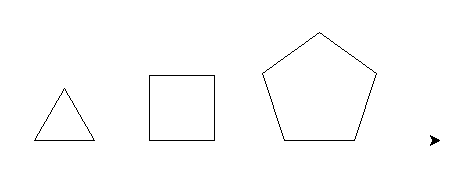# Functions with parameters¶

## Introduction¶

As we shrink down our code and add functions to remove duplication, we are factorizing it. This is a good thing to do. But the functions we have defined so far are not very flexible. The variables are defined inside the function, so if we want to use a different angle or a distance then we need to write a new function. Our hexagon function can only draw one size of hexagon!

That is why we need to be able to give parameters, also called arguments, to the function. This way the variables in the function can have different values each time we call the function:

Remember how we defined the function `line_without_moving()` in the previous section:

```def line_without_moving():
turtle.forward(50)
turtle.backward(50)
```

We can improve it by giving it a parameter:

```def line_without_moving(length):
turtle.forward(length)
turtle.backward(length)
```

The parameter acts as a variable only known inside the function’s definition. We use the newly defined function by calling it with the value we want the parameter to have like this:

```line_without_moving(50)
line_without_moving(40)
```

We have been using functions with parameters since the beginning of the tutorial with `turtle.forward()`, `turtle.left()`, etc...

And we can put as many arguments (or parameters) as we want, separating them with commas and giving them different names:

```def tilted_line_without_moving(length, angle):
turtle.left(angle)
turtle.forward(length)
turtle.backward(length)
```

## A parameterized function for a variable size hexagon¶

### Exercise¶

Write a function that allows you to draw hexagons of any size you want, each time you call the function.

### Solution¶

```def hexagon(size):
for _ in range(6):
turtle.forward(size)
turtle.left(60)
```

## A function of several parameters¶

### Exercise¶

Write a function that will draw a shape of any number of sides (let’s assume greater than two) of any side length. Get it to draw some different shapes.

Here’s an example of drawing shapes with this function:Tip

The sum of the external angles of any shape is always 360 degrees!

### Solution¶

```def draw_shape(sides, length):
for _ in range(sides):
turtle.forward(length)
turtle.right(360 / sides)
```

### Bonus¶

It might sound crazy, but it’s perfectly possible to pass a function as a parameter to another function! Python regards functions as perfectly normal ‘things’, the same as variables, numbers and strings.

For instance, you could create a shape drawing function which turned one way or another depending on which turtle function you passed to it - `turtle.left` or `turtle.right`.

See if you can implement this!

Note

Passing a function (e.g `turtle.left`) is different than calling it, which would instead be written `turtle.left(45)`.